# Starting with a capacitor charged to 30V, how long will it take to completely discharge it?...

Starting with a capacitor charged to 30V, how long will it take to completely discharge it?

a.) 30 sec

b.) 10 sec

c.) The capacitor would technically never discharge completely

d.) Not enough information is given to answer this question

Since the capacitor discharge equation is given by

Vt = Vo (e-t/RC)

For fully discharging the capacitor,

Vt = 0

=> 0 = Vo (e-t/RC)

Vo = 30V

=>e-t/RC = 0

This is only possible when,

t/RC ->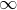=> RC -> 0

Therefore we can say that the capacitor would never technically fully discharge

Hence,

Correct option is C

#### Earn Coin

Coins can be redeemed for fabulous gifts.

Similar Homework Help Questions
• ### How long does it take to discharge a capacitor via steady current?

A 2000 microfarad capacitor, initially charged to 100 volts, is discharged by a steady current of 100 microamps. How long does it take to discharge the capacitor to 0volts?

• ### A 10?F capacitor initially charged to 30?C is discharged through a 1.3k? resistor. How long does...

A 10?F capacitor initially charged to 30?C is discharged through a 1.3k? resistor. How long does it take to reduce the capacitor's charge to 3.0?C ? Answer should be in ms

• ### You have a 2.0-uF capacitor and a 9.0-V battery: 2a. If initially uncharged, how long does...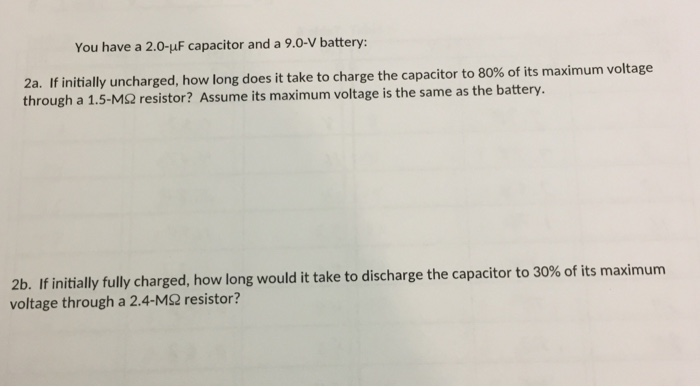You have a 2.0-uF capacitor and a 9.0-V battery: 2a. If initially uncharged, how long does it take to charge the capacitor to 80% of its maximum voltage through a 1.5-M2 resistor? Assume its maximum voltage is the same as the battery. 2b. If initially fully charged, how long would it take to discharge the capacitor to 30% of its maximum voltage through a 2.4-MΩ resistor?

• ### (30 points) 4) A 10.0-uF capacitor is charged though a 50,000-2 resistor. (a)How long does it...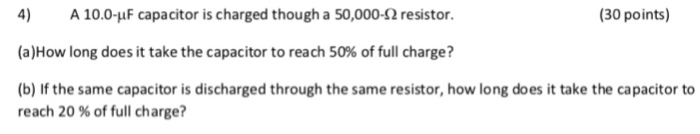(30 points) 4) A 10.0-uF capacitor is charged though a 50,000-2 resistor. (a)How long does it take the capacitor to reach 50% of full charge? (b) If the same capacitor is discharged through the same resistor, how long does it take the capacitor to reach 20 % of full charge?

• ### Question 2 A 7.35 uF capacitor is charged to 1.23x103 V and is then connected in...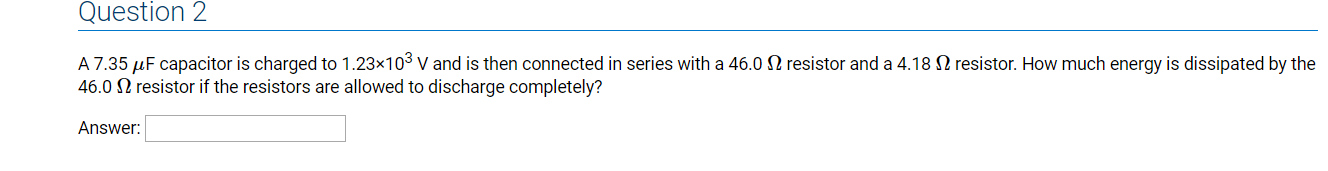Question 2 A 7.35 uF capacitor is charged to 1.23x103 V and is then connected in series with a 46.0 32 resistor and a 4.18 12 resistor. How much energy is dissipated by the 46.0 12 resistor if the resistors are allowed to discharge completely? Answer:

• ### 21.  Look at Figure 30-26 and you will see a capacitor charge and discharge curve...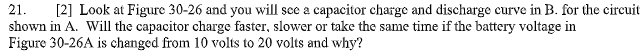21.  Look at Figure 30-26 and you will see a capacitor charge and discharge curve in B. for the circuit shown in A. Will the capacitor charge faster, slower or take the same time if the battery voltage in Figure 30-26A is changed from 10 volts to 20 volts and why? 1 time-constant period), the voltage act switched to DISCHARGE, the voltage Example 30-6 Assume a de voltage source of 100 Vis applied to a series RC circuit consisting...

• ### Answer question A and B a) how much energy is stored in the defibrillators capacitor B)...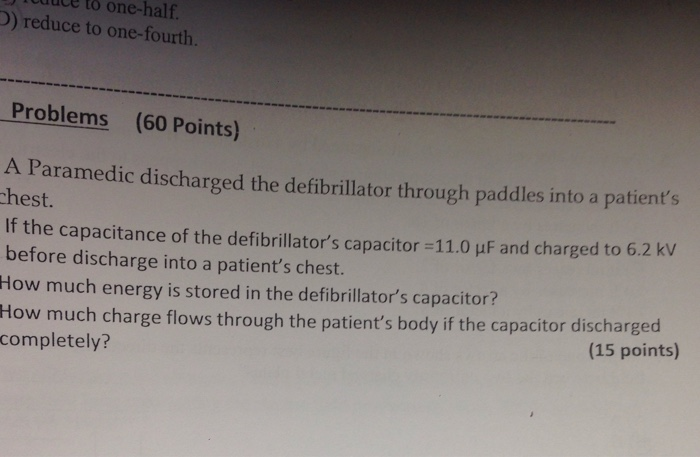Answer question A and B a) how much energy is stored in the defibrillators capacitor B) how much charge flows through the patients body If the capacitor discharged completely? to one-half. D) reduce to one-fourth. Problems (60 Points) A Paramedic discharged the defibrillator through paddles into a patient's hest. If the capacitance of the defibrillator's capacitor-11.0 μF and charged to 62 kV before discharge into a patient's chest. How much energy is stored in the defibrillator's capacitor? Ho w much...

• ### A 20 μF capacitor initially charged to 25 μC is discharged through a 1.5 kΩ resistor. Part A How long does it take to r...

A 20 μF capacitor initially charged to 25 μC is discharged through a 1.5 kΩ resistor. Part A How long does it take to reduce the capacitor's charge to 10 μC?

• ### Figure 20.47 shows how a bleeder resistor (R = 260 kohm) is used to discharge a...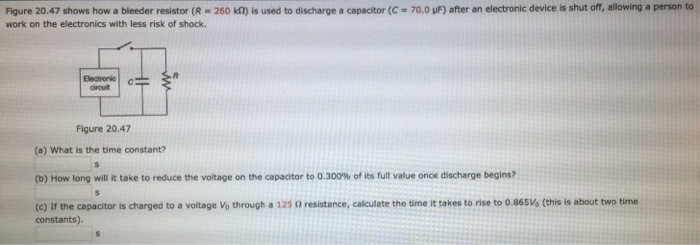Figure 20.47 shows how a bleeder resistor (R = 260 kohm) is used to discharge a capacitor (c = 70.mu F) after an electronic device is shut off, allowing a person to work on the electronics with less risk of shock. (a) What is the time constant? s (b) How long will it take to reduce the voltage on the capacitor to o.300% of its full value once discharge begins? s (c) If the capacitor is charged to a voltage...

• ### The figure below shows how a bleeder resistor (R = 226 kil) is used to discharge...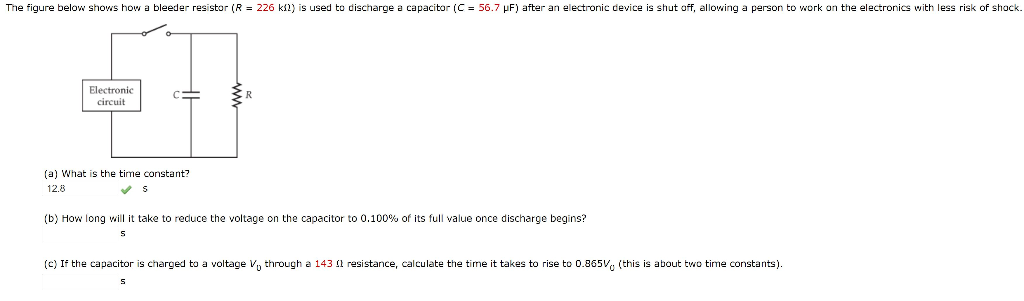The figure below shows how a bleeder resistor (R = 226 kil) is used to discharge a capacitor (C = 56.7 PF) after an electronic device is shut off, allowing a person to work on the electronics with less risk of shock Electronic circuit CE (a) What is the time constant? 12.8 (b) How long will it take to reduce the voltage on the capacitor to 0.100% of its full value once discharge begins? (c) If the capacitor is charged...